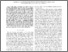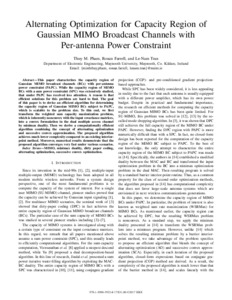# Alternating Optimization for Capacity Region of Gaussian MIMO Broadcast Channels with Per-Antenna Power Constraint

Pham, Thuy M. and Farrell, Ronan and Tran, Le-Nam (2017) Alternating Optimization for Capacity Region of Gaussian MIMO Broadcast Channels with Per-Antenna Power Constraint. In: 2017 IEEE 85th Vehicular Technology Conference (VTCSpring) : Proceedings. Institute of Electrical and Electronics Engineers, Red Hook, NY, USA. ISBN 978-1-5090-5932-4Previewmore...Add this article to your Mendeley library

## Abstract

This paper characterizes the capacity region of Gaussian MIMO broadcast channels (BCs) with per-antenna power constraint (PAPC). While the capacity region of MIMO BCs with a sum power constraint (SPC) was extensively studied, that under PAPC has received less attention. A reason is that efficient solutions for this problem are hard to find. The goal of this paper is to devise an efficient algorithm for determining the capacity region of Gaussian MIMO BCs subject to PAPC, which is scalable to the problem size. To this end, we first transform the weighted sum capacity maximization problem, which is inherently nonconvex with the input covariance matrices, into a convex formulation in the dual multiple access channel by minimax duality. Then we derive a computationally efficient algorithm combining the concept of alternating optimization and successive convex approximation. The proposed algorithm achieves much lower complexity compared to an existing interiorpoint method. Moreover, numerical results demonstrate that the proposed algorithm converges very fast under various scenarios.

Item Type: Book Section This paper was presented at 2017 IEEE 85th Vehicular Technology Conference (VTCSpring), Sydney, Australia, 4–7 June 2017. MIMO; Optimization; Covariance matrices; Complexity theory; Algorithm design and analysis; Antennas; Standards; antenna arrays; approximation theory; broadcast channels; channel capacity; concave programming; convex programming; covariance matrices; duality (mathematics); Gaussian channel; MIMO communication; minimax techniques; multi-access systems; alternating optimization; Gaussian MIMO broadcast channels; per-antenna power constraint; PAPC; sum power constraint; SPC; interiorpoint method; successive convex approximation; nonconvex problem; minimax duality; convex formulation; input covariance matrices; SPC; MIMO BCs capacity region; dual multiple access channel; weighted sum capacity maximization problem; Gaussian MIMO BCs subject; Faculty of Science and Engineering > Electronic EngineeringFaculty of Science and Engineering > Research Institutes > Hamilton Institute 11913 https://doi.org/10.1109/VTCSpring.2017.8108405 Ronan Farrell 28 Nov 2019 16:44 Institute of Electrical and Electronics Engineers YesItem control page